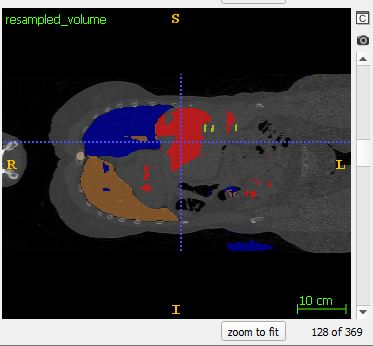# 3D UNet Patch-Based Segmentation Output Artifacts

Hi All,

I’m having some issues using a 3D UNet (base 32, depth 4) for multi-organ segmentation. Due to memory constraints, I use 128x128,128 patches with a sliding window, with overlap of 32 in each axis. I use a combined loss of weighted DICE and weighted CE, an adam optimizer with lr=0.00001. The network has learned something, and results (as you’ll see) look…interesting.

The following inference images come from a similar patch-based approach. To avoid discontinuities at the edges, I sample the volume with a sliding window larger than the area of interested, and crop the 128x128x128 output that I’m interested in from the output of this larger sliding window. In this way, each sample contains more context from the volume to smooth the output between subsequent samples.

Despite this approach, I’m still finding discontinuities at the edges of each sample. Note: I grab the 128x128x128 center of each larger sliding window. The following images come from the output of coronal slice 128, and coronal slice 129: which is the border between subsequent samples.Moreover, blocky behavior (Also noted here, but unfortunately never answered: How to avoid 'blocky' output when stitching 3D patches back together?)
can also be witnessed in other slices. Here particularly in the lung and liver segmentations :

Anyone have experience with this problem that can give some advice would be greatly appreciated!

The blocky or patchy approach is not a pytorch issue, from my understanding it is caused by one of the two reasons, either your network does not have enough context to work with i.e. input block size is too small or your code to stitch them back together is messing up somehow.

The code mentioned in the lined question of @farazk86 should work well for stitching the patches back together without any block artefacts. You should have a similar code, copied here for easy reference:

``````def unpad(patch, index, shape, pad_width=4):
"""
Remove `pad_width` voxels around the edges of a given patch.
"""

def _new_slices(slicing, max_size):
if slicing.start == 0:
p_start = 0
i_start = 0
else:

if slicing.stop == max_size:
p_stop = None
i_stop = max_size
else:

return slice(p_start, p_stop), slice(i_start, i_stop)

D, H, W = shape

i_c, i_z, i_y, i_x = index
p_c = slice(0, patch.shape)

p_z, i_z = _new_slices(i_z, D)
p_y, i_y = _new_slices(i_y, H)
p_x, i_x = _new_slices(i_x, W)

patch_index = (p_c, p_z, p_y, p_x)
index = (i_c, i_z, i_y, i_x)
return patch[patch_index], index
``````

If stitching is not your problem them look into increasing the block size.

P.S. I;m very interested in your mentioned approach:

I use a combined loss of weighted DICE and weighted CE

Can you link this being used somewhere I would like to see it in action. or explain the implementation

Thanks

Hi,

Thanks for the tip. I’ll look into the stitching and see if that’s the problem. In the meantime, the weighted DICE and weighted cross entropy I implemented in the following manner:

Because it’s a highly unbalanced dataset (21 organs, some of them much smaller than others), we calculate the weights online, by passing the ground truth to the following function:

``````    def compute_weights(self, y):
w = np.zeros(self.num_classes)
labels, histo = np.unique(y.numpy(), return_counts=True)

#index=0
#for x in np.nditer(labels):
#   w[x]= histo[index]
#   index+=1
# for i in range(len(labels)):
#     if i in labels:
#         w[labels[i]] = histo[index]
#         index+=1
# w[w==0]= eps
freq = histo / np.sum(histo)
med_freq = np.median(freq)
weights = med_freq / (freq+eps)
for i, label in enumerate(weights):
w[int(labels[i])] = weights[i]

``````

When backpropagating loss, I figured that it doesn’t make much sense to backpropagate error for classes that don’t exist in the given patch, so we start with all weights equaling zero, and only change those weights in the following manner (median frequency balacning) for those classes existing in the current patch.

Then one can give these weights to torch.nn.CrossEntropyLoss to calculate the weighted CE:

``````        self.cross_entropy_loss = nn.CrossEntropyLoss(weight=weight)
CE_Loss = self.cross_entropy_loss.forward(input, target)
``````

The dice loss we calculate via the dice score, and then scale the loss for each channel by the online weights for the given patch : weights* (1 - Dice_Score).

Before calculating these median freq weights, my network wouldn’t learn from precalculated weights on the entire volume. This is because a patch was not representative of the volume, and so we were getting a lot of error backpropagation for classes that weren’t even represented in the current patch.

Hope this makes sense! If you have any feedback, or I missed something, lemme know!

1 Like